Scattering and tunnelling

Start this free course now. Just create an account and sign in. Enrol and complete the course for a free statement of participation or digital badge if available.

Free course

# 3.3 Scattering from a finite square step

The kind of one-dimensional scattering target we shall be concerned with in this section is called a finite square step. It can be represented by the potential energy function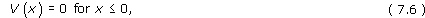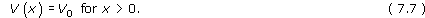The finite square step (Figure 8) provides a simplified model of the potential energy function that confronts an electron as it crosses the interface between two homogeneous media. The discontinuous change in the potential energy at x = 0 is, of course, unrealistic, but this is the feature that makes the finite square step simple to treat mathematically. The fact that we are dealing with a square step means that we shall only have to consider two regions of the x-axis: Region 1 where x ≤ 0, and Region 2 where x > 0.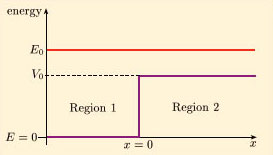Figure 8 A finite square step of height V0 < E0

Classically, when a finite square step of height V0 scatters a rightward moving beam in which each particle has energy E0 > V0, each of the particles will continue moving to the right but will be suddenly slowed as it passes the point x = 0. The transmitted particles are slowed because, in the region x > 0, each particle has an increased potential energy, and hence a reduced kinetic energy. The intensity of each beam is the product of the linear number density and the speed of the particles in that beam. To avoid any accumulation of particles at the step, the incident and transmitted beams must have equal intensities; the slowing of the transmitted beam therefore implies that it has a greater linear number density than the incident beam.

## Exercise 2

In general terms, how would you expect the outcome of the quantum scattering process to differ from the classical outcome?

In view of the quantum behaviour of individual particles (as represented by wave packets) when they meet a finite square barrier, it is reasonable to expect that there is some chance that the particles encountering a finite square step will be reflected. In the case of quantum scattering we should therefore expect the outcome to include a reflected beam as well as a transmitted beam, even though E0 > V0.

We start our analysis by writing down the relevant Schrödinger equation: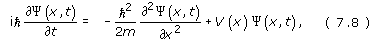where V(x) is the finite square step potential energy function given in Equations 7.6 and 7.7. We seek stationary-state solutions of the form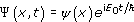, where E0 is the fixed energy of each beam particle. The task of solving Equation 7.8 then reduces to that of solving the time-independent Schrödinger equations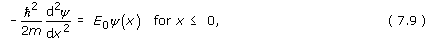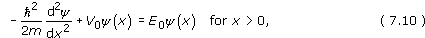A simple rearrangement gives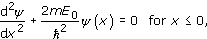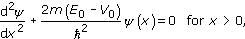and it is easy to see that these equations have the general solutions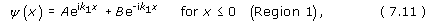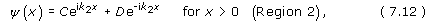where A, B, C and D are arbitrary complex constants, and the wave numbers in Region 1 and Region 2 are respectively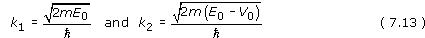You may wonder why we have expressed these solutions in terms of complex exponentials rather than sines and cosines (recall the identity eix = cos x + i sin x). The reason is that the individual terms in Equations 7.11 and 7.12 have simple interpretations in terms of the incident, reflected and transmitted beams. To see how this works, it is helpful to note that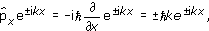where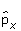is the momentum operator in the x direction.

It therefore follows that terms proportional to eikx are associated with particles moving rightward at speed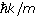, while terms proportional to e−ikx are associated with particles moving leftward at speed.

These directions of motion can be confirmed by writing down the corresponding stationary-state solutions, which take the form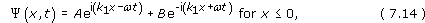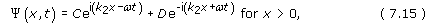where ω = E0/. We can then identify terms of the form ei(kxωt) as plane waves travelling in the positive x-direction, while terms of the form e−i(kx+ωt) are plane waves travelling in the negative x-direction. None of these waves can be normalised, so they cannot describe individual particles, but you will see that they can describe steady beams of particles.

In most applications of wave mechanics, the wave function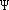(x, t) describes the state of a single particle, and |(x, t)|2 represents the probability density for that particle. In the steady-state approach to scattering, however, it is assumed that the wave function(x, t) describes steady beams of particles, with |(x, t)|2 interpreted as the number of particles per unit length – that is, the linear number density of particles. We know that the wave function is not normalisable, and this corresponds to the fact that the steady beams extend indefinitely to the left and right of the step and therefore contain an infinite number of particles. This will not concern us, however, because we only need to know the linear number density of particles, and this is given by the square of the modulus of the wave function.

Looking at Equation 7.14, and recalling that the first term Aei(k1xωt) represents a wave travelling in the positive x-direction for x ≤ 0, we identify this term as representing the incident wave in Region 1 (x ≤ 0). We can say that each particle in the beam travels to the right with speed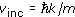, and that the linear number density of particles in the beam is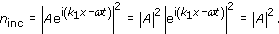(You will find further justification of this interpretation in Section 3.4.)

Similarly, the second term on the right of Equation 7.14 can be interpreted as representing the reflected beam in Region 1 (x ≤ 0). This beam travels to the left with speed vref =k1/m and has linear number density nref = |B|2.

The first term on the right of Equation 7.15 represents the transmitted beam in Region 2 (x > 0). This beam travels to the right with speed vtrans =k2/m and has linear number density ntrans = |C|2. The second term on the right of Equation 7.15 would represent a leftward moving beam in the region x > 0. On physical grounds, we do not expect there to be any such beam, so we ensure its absence by setting D = 0 in our equations.

Using these interpretations, we see that the beam intensities are: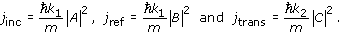Expressions for the reflection and transmission coefficients then follow from Equation 7.5: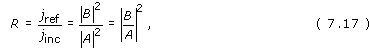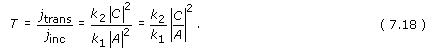It is worth noting that the expression for the transmission coefficient includes the wave numbers k1 and k2, which are proportional to the speeds of the beams in Regions 1 and 2. The wave numbers cancel in the expression for the reflection coefficient because the incident and reflected beams both travel in the same region.

To calculate R and T, we need to find the ratios B/A and C/A. To achieve this, we must eliminate unwanted arbitrary constants from our solutions to the time-independent Schrödinger equation. This can be done by requiring that the solutions satisfy continuity boundary conditions:

•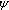(x) is continuous everywhere.

• d(x)/dx is continuous where the potential energy function is finite.

The first of these conditions tells us that our two expressions for(x) must match at their common boundary x = 0. From Equations 7.11 and 7.12, we therefore obtain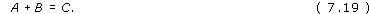Taking the derivatives of Equations 7.11 and 7.12,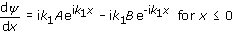so requiring the continuity of d/dx at x = 0 implies that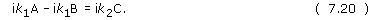After some manipulation, Equations 7.19 and 7.20 allow us to express B and C in terms of A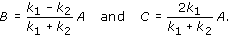Combining these expressions with Equations 7.17 and 7.18, we finally obtain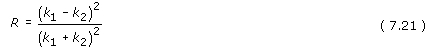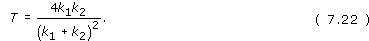Since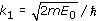and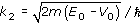, where E0 is the incident particle energy and V0 is the height of the step, we have now managed to express R and T entirely in terms of given quantities. The transmission coefficient, T, is plotted against E0/V0 in Figure 9.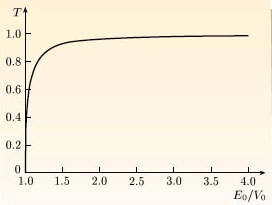Figure 9 A graph of the transmission coefficient T against E0/V0 for a finite square step of height V0

The above results have been derived by considering a rightward moving beam incident on an upward step of the kind shown in Figure 8. However, almost identical calculations can be carried out for leftward moving beams or downward steps. Equations 7.21 and 7.22 continue to apply in all these cases, provided we take k1 to be the wave number of the incident beam and k2 to be the wave number of the transmitted beam.

The formulae for R and T are symmetrical with respect to an interchange of k1 and k2, so a beam of given energy, incident on a step of given magnitude, is reflected to the same extent no matter whether the step is upwards or downwards. This may seem strange, but you should note that the reflection is a purely quantum effect, and has nothing to do with any classical forces provided by the step.

Another surprising feature of Equation 7.21 is that R is independent of m and so does not vanish as the particle mass m becomes very large. However, we know from experience that macroscopic objects are not reflected by small changes in their potential energy function – you can climb upstairs without serious risk of being reflected! How can such everyday experiences be reconciled with wave mechanics?

This puzzle can be resolved by noting that our calculation assumes an abrupt step. Detailed quantum-mechanical calculations show that Equation 7.21 provides a good approximation to reflections from a diffuse step provided that the wavelength of the incident particles is much longer than the distance over which the potential energy function varies. For example, Equation 7.21 accurately describes the reflection of an electron with a wavelength of 1 nm from a finite step that varies over a distance of order 0.1 nm. However, macroscopic particles have wavelengths that are much shorter than the width of any realistic step, so the above calculation does not apply to them. Detailed calculations show that macroscopic particles are not reflected to any appreciable extent so, in this macroscopic limit, quatum mechanics agrees with both classical physics and everyday experience.

Although we have been discussing the behaviour of beams of particles in this section, it is important to realise that these beams are really no more than a convenient fiction. The beams were simply used to provide a physical interpretation of de Broglie waves that could not be normalised. The crucial point is that we have arrived at explicit expressions for R and T, and we have done so using relatively simple stationary-state methods based on the time-independent Schrödinger equation rather than computationally complicated wave packets. Moreover, as you will see, the method we have used in this section can be generalised to other one-dimensional scattering problems.

## Exercise 3

• (a) Use Equations 7.21 and 7.22 to show that R + T = 1.

• (b) Evaluate R and T in the case that E0 = 2V0, and confirm that their sum is equal to 1 in this case.

• (a) From Equations 7.21 and 7. 22,

•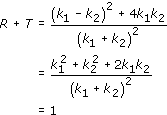• (b) From Equation 7.13, we have

•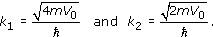• So, in this case,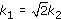. Therefore

•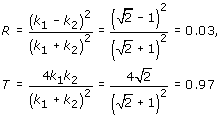• So we can check that R + T = 1 in this case.

## Exercise 4

Consider the case where k2 = k1/2.

• (a) Express B and C in terms of A.

• (b) Show that in the region x > 0, we have ||2 = 16|A|2/9 = constant, while in the region x ≤ 0, we have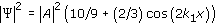.

• (c) What general physical phenomenon is responsible for the spatial variation of ||2 to the left of the step?

• (d) If the linear number density in the incident beam is 1.00 × 1024 m−1, what are the linear number densities in the reflected and transmitted beams?

• (a) When k2 = k1/2, we have

•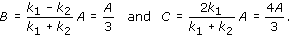• (b) From Equation 7.15 with D = 0,

•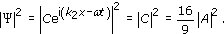• Similarly, from Equation 7.14,

•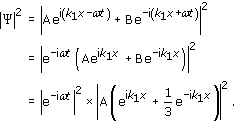• Since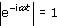, we have

•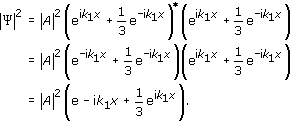• Multiplying out the brackets, we find

•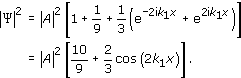• (c) The variation indicated by the cosine-dependence to the left of the step is a result of interference between the incident and reflected beams. The presence of interference effects was noted earlier when we were discussing the scattering of wave packets but there the effect was transitory. In the stationary-state approach interference is a permanent feature.

• (d) The linear number densities in the incident, reflected and transmitted beams are given by |A|2, |B|2 and |C|2. The question tells us that |A|2 = 1.00 × 1024 m−1, so the linear number density in the reflected and transmitted beams are

•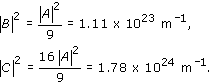• Note that the transmitted beam is denser than the incident beam: |C|2 > |A|2. However, since k2 = k1/2, we have jtrans < jinc. The transmitted beam is less intense than the incident beam because it travels much more slowly.

## Exercise 5

Based on the solution to Exercise 4, sketch a graph of ||2 that indicates its behaviour both to the left and to the right of a finite square step.

A suitable graph is shown in Figure 10.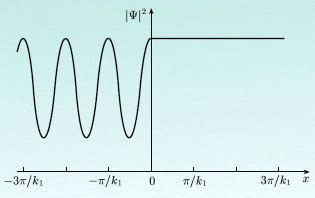Figure 10 |Ψ|2 plotted against x for a finite square step at x = 0 when E0 > V0
SM358_1